Mobile QR Code1. (1School of Comp. & Info. Engineering, Kwangwoon University, Kwangwoon-ro 20, Nowon-gu, Seoul 01897, Korea)
2. (School of Electronic and Electrical Engineering, Hongik University, Wausan-ro 94, Mapo-gu, Seoul 04066, Korea)

Approximate computing, stochastic computing, fully connected mesh network; cube network, energy efficiency, edge detection

## I. INTRODUCTION

Research into high density and low power solutions has garnered significant interest in various alternative computing technologies. One of these alternatives is low-cost and energy-efficient hardware that can be used to perform low-precision calculations with approximate computing. Because the area and power of a computing device increases according to the bit width, energy can be efficiently used within the same area and power range through approximate computing [1-4].

Stochastic computing (SC), an approximate computing method, has recently attracted attention owing to its compact size, low power, and soft error tolerance. SC uses unary-encoded bitstreams that are represented by 0 and 1 over time, unlike binary-encoded calculations. Hence, SC is suitable for applications such as image processors, digital filters, neural networks, and low-density parity check (LDPC) [5-18].

In SC, the value of a bitstream is encoded as the number of components by 0 and 1, and the precision of the bitstream is determined by the bitstream length. Unlike binary-encoded calculations, many operations in SC do not produce accurate results because the correlation between the input operands is insufficient or excessive. For example, SC subtraction or division requires a positive correlation in the inputs, thus insufficient correlation causes problems (10). In addition, these correlations caused by incorrect SC input operands increase errors. Although SC operation circuit works accurately, its results are different depending on correlated or uncorrelated inputs [13-18].

In SC, uncorrelated stochastic numbers (SNs) are required for better (i.e., more accurate) results in addition (using MUX) and multiplication (using AND gate). Uncorrelation can be usually achieved by using independent (or separated) two random number generators (RNGs). However, they require additional area.

Herein, we present a design for generating high-quality bitstreams for a more accurate operation of SC. In the proposed design, an RNG is shared with several comparators that generate stochastic numbers such that it can reduce the total size of the stochastic number generator (SNG) without losing accuracy in SC. This sharing method exchanges the output of the RNG based on a fully connected cube network to reduce the correlation between stochastic numbers. By applying the proposed method to an image processor, the area is reduced, and errors are minimized compared with conventional designs.

This letter is organized as follows: Section II provides background regarding SC. Section III presents our new SNG and improved SC operation circuits. In Section IV, comparison edge detection using an accurate operator and a stochastic operator in the real world is evaluated. Section V presents the conclusions.

## II. PRELIMINARY

SC was introduced in the 1960s, and bitstream (or stochastic number) is represented by a stream of bits whose probability of occurrence matches the number to be computed (5). An input number X with L bits can be expressed as $S_{x}=s_{\mathrm{x} 1} \mathrm{~s}_{\mathrm{x} 2} \cdots \quad s_{\mathrm{xL}}$.

Bitstream is encoded by unipolar and bipolar coding formats for SC. Both of these coding formats have the same sequence and can coexist within a system. Bipolar formats can handle negative numbers differently from unipolar formats. When the bitstream length, L is the same, the accuracy of the bipolar format is half that of the unipolar format. The encoded value is the sum of each position in the bitstream divided by the length of bitstream L and the range is (0,1) in the unipolar format. The weight of 1 is “+1” and the weight of 0 is “0.” For example, when there are three “1s” and the bitstream length is 8, the bitstream A = 00010011 encodes the value PA = 0.375, which is able to present the range of [0, +1] in the unipolar format. In contrast, it is possible to express the range of [-1, +1] in the bipolar format. And it can encode negative and positive numbers because the weight of 1 is “+1” and 0 is “-1”. For instance, the same bitstream used above A = 00010011 can encode the value $P_{A} = -0.25$.

SC offers the advantages of compact size, low power, and simple logic circuit using bitstreams for complex arithmetic operations. For instance, when two bitstreams A = 01010101 and B = 11111100 exist, they encode $P_{A}$ = 0.5 and $P_{B}$ = 0.75, respectively, and multiplication can be performed by calculating the bit-wise AND of A and B. Therefore, Result = 01010100 and $P_{Result}$ is 0.375. A multiplexer is used to scale addition in SC because a multiplexer has a selection function. When there are two input data, A and B, and a single control input, S, bitstreams $P_{A}$, $P_{B}$, and $P_{S}$ are sequentially supplied to these inputs, respectively. The result of the multiplexer, $P_{Result}$, is (1 - $P_{S}$) × $P_{A}$ + $P_{S}$ × $P_{B}$. Furthermore, SC can calculate mathematical functions such as tanh or absolute subtraction using XOR.

The correlation between bitstreams generated by SNG affects the accuracy of SC. The correlation between the two bitstreams has been defined as stochastic computing correlation (SCC) (13). SCC is based on the product of the number of bits at the same position of two bitstreams, and the value of SCC becomes larger as the number of these overlapping bits increases. The difference is normalized between [-1, +1] when dividing by the maximum or minimum difference. No correlation exists between two bitstreams when SCC = 0. Furthermore, SCC = +1 means that a maximum correlation exists; meanwhile, SCC = -1 means a minimum correlation.

The accuracy of SC depends on the bitstream that can be used in the operation, i.e., the sequence of the bitstreams. To generate such a bitstream, an SNG is required to convert from an input number to bitstreams. A conventional SNG is based on a linear-feedback shift register (LFSR) that generates a random number during a given period and a comparator that compares an input number and a random number from the LFSR, as shown in Fig. 1. In this figure, the comparator receives a random value from the 8-bit LFSR and input number x; subsequently, these two values are compared. It then outputs one bit of the stochastic number bit sxi, which is 0 or 1, corresponding to the LFSR's cycle. When the value generated by the LFSR is less than the input value x, the output is 1 or 0 otherwise. The LFSR has a structure in which the value input to the register is computed as a linear function of the previous state values. The n-bit LFSR applies the value of 1 bit to 2n-th bit to the specified polynomial, which outputs repeatedly for a specific period. The run time in SC is determined by the length of the bitstream. It is noteworthy that SC requires a clock of 2n to convert an integer of n bits to bitstreams; the larger the bitstream length, the more time and energy are consumed.

Fig. 1. Conventional Stochastic number generator: 8-bit linear-feedback shift register (LFSR) and comparator.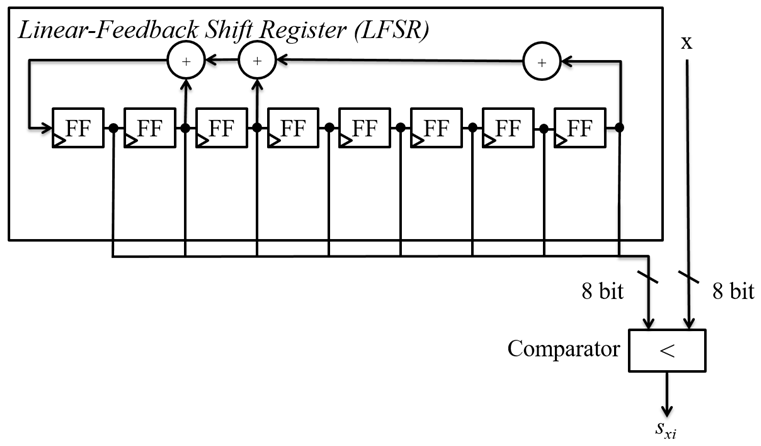Fig. 2. Various stochastic number generation methods (a) dedicated LFSR, (b) sharing, (c) sharing with an inverter, (d) proposed method.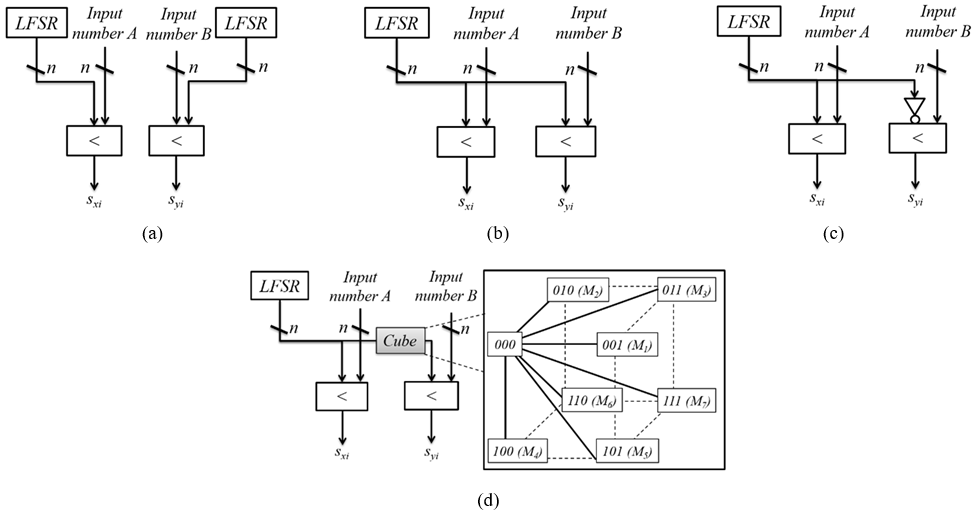## III. STOCHASTIC NUMBER GENERATION BASED ON FULLY-CONNECTED CUBE NETWORK

### 1. Basic Structures

One of the advantages of SC is that it can be implemented as a simple circuit for complex operations. However, the need for a large number of SNGs, which occupies a large area in SC, is in violation of SC's orientation in terms of power and area. For instance, image processor based on SC requires many SNGs, which constitutes more than 80% of the total circuit for image frames (14). In addition, when generating bitstreams, sharing the LFSR or attaching the inverter of the output of the LFSR cannot provide accurate results compared with using various LFSRs (15). Fig. 2 shows various SNG schemes: (a) dedicated LFSRs are used for each input (8), (b) one LFSR is shared (12), (c) an inverter is used with one LFSR when converting operands with stochastic numbers (14) and (d) one LFSR is shared with a fully-connected cube network, which is our proposed scheme. Therefore, reducing the area of the SNG that generates the correct bitstream is important in SC.

Fig. 3. Various network architecture (a) partial mesh, (b) fully connected mesh, (c) cube network, (d) fully connected cube network (the proposed method).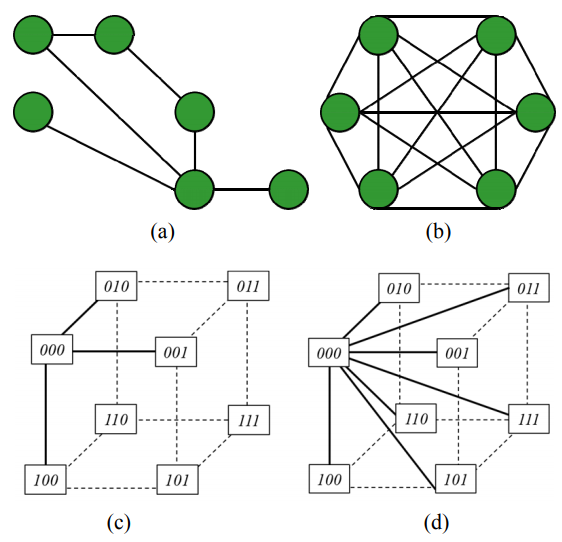Sharing LFSRs with other comparators has been considered to reduce the hardware overhead of SNGs (16). This allows K SNGs to share only one LFSR and reduce the area to 1/k. In addition, sharing an LFSR with an inverter has been proposed. However, the value of SCC was either the maximum or converged to the minimum, respectively.

We propose generating enhanced bitstreams and reducing hardware size, by changing the output of the LFSR based on the fully connected cube network with a mesh architecture. The mesh architecture, which is a kind of network typology, is typically used in public data communication networks (19). This is distinguished by a partial mesh with only some nodes connected, as shown in Fig. 3(a) and a fully connected network with all nodes connected, as shown in Fig. 3(b).

The existing n-cube network, also called hypercube, is an interconnect function with $n = log_2N$. n is called the dimension of the n-cube network and N means the total number of nodes (20,21). When the node addresses are considered as the corners of an n-dimension cube, the network connects each node to its n neighbors. In an n cube, individual nodes are uniquely identified by n-bit addresses ranging from 0 to N-1. Given a node with binary address b, this node is connected to all nodes whose binary addresses differ from b by exactly one bit and it is defined as follows:

$C_{i}\left(b_{n-1} b_{n-2} \cdots b_{i} \cdots b_{1} b_{0}\right)=b_{n-1} b_{n-2} \cdots b_{i}^{\prime} \cdots b_{1} b_{0}$ $\left(n=\log _{2} N, 0 \leq i<n\right)$

For instance, in a 3-cube network, in which eight nodes exist, node 000$_2$ (0) is connected to nodes 001$_2$ (1), 010$_2$ (2), and 100$_2$ (4), as shown in Fig. 3(c).

Assume that the node of the n-cube network is a fully connected mesh with each neighbor. Given a node with a binary address b, this node is connected to all nodes except itself; therefore, the ith mesh (Mi) is defined as follows:

$M_{i}\left(b_{n-1} b_{n-2} \cdots b_{i} b_{i-1} \cdots b_{1} b_{0}\right)$$=M_{j}\left(b_{n-1} b_{n-2} \cdots b_{j}^{\prime} b_{j-1}^{\prime} \cdots b_{1} b_{0}\right)\left\{\begin{array}{c}i f\left(k_{m}=1\right), b_{j}=b_{i}^{\prime} \\ e l s e b_{j}=b_{i}\end{array}\right. \left(n=\log _{2} N, 1 \leq i, j<N, i_{10}=\sum_{m=0}^{n-1} k_{m} \times 2^{m}\right) For example, in a 3-cube network with eight nodes, node 000_2 (0) is connected to nodes between 001_2 (1) and 111_2 (7), as shown in Fig. 3(d). Assume that b is the output number from the LFSR and uses a modified random number for stochastic number generation. For instance, we can use each of the different eight outputs for stochastic number generation when one LFSR with an 8-bit output exists by selecting one according to Mi formula. This is shown in Table 1. The diagram of this design is shown in Fig. 2(d). In this design, the output of the LFSR is exchanged by a fully connected cube network and it is connected to the comparator, while the output of the LFSR is fed to another comparator with no exchange. Compared to sharing an LFSR, this technique does not require any hardware overhead because it just inverts the output bit address from a random number generator by selecting one according to M formula. ### 2. Simulation Results We synthesized the system using Quartus (22) and Verilog HDL to compare the error characteristics of the adder and multiplier through various SNGs. To verify operation errors, 100,000 randomly generated 8-bit numbers were used. The average SCC was calculated by . The error distance (ED) is S C C_{\text {arg}}=\frac{\sum_{i=0}^{N} S C C\left(X_{i}, Y_{i}\right)}{N} defined as ED = |ACC-APP|, where ACC is the result by the obtained accurate operator, and APP is the result of the stochastic operator; the relative error (RE) is calculated by R E=\frac{E D}{A C C} . The RE histogram of each adder is shown in Fig. 4(a), and the results of the multipliers are presented in Fig. 4(b). Compared with the conventional method, the average SCC, maximum ED, average absolute RE, and standard deviation (SD) were enhanced by the proposed design, as shown in Table 2 and Fig. 4. The average SCC for the inverting method is close to -1, which implies a highly negative correlation. Furthermore, the average SCC for the sharing is 1, which indicates a highly positive correlation. However, the average SCC for the dedicated method and the average of the proposed method is half the SCC in the sharing method. It is noteworthy that the proposed method does not require any overhead compared with the dedicated method. In the SC adder, the proposed structure (M_{2}) exhibits a reduced average ED (AVG_ED) by 29%, maximum ED (MAX_ED) by 37%, average absolute RE (AVG_ABS_RE) by 25%, and SD by 15% compared with the inverter method. Furthermore, the multiplier based on the proposed structure (M_{2}) has an improved RE AVG by 3× and SD by 20% compared with the sharing method. Table 1. Order of eight different outputs with three bits according to fully connected cube network function  b_2b_1b_0 M1(b_2b_1b_0) = b_2$$b_1$b$^2$$_0 M2(b_2b_1b_0) = b_2b^2$$_1$$b_0 M3(b_2b_1b_0) = b_2b^2$$_1$b$^2$$_0 M4(b_2b_1b_0) = b^2$$_2$$b_1$$b_0$ M5($b_2b_1b_0$) = b$^2$$_2$$b_1$b$^2$$_0 M6(b_2b_1b_0) = b^2$$_2$b$^2$$_1$$b_0$ M7($b_2b_1b_0$) = b$^2$$_2b^2$$_1$b$^2$$_0$ 000$_2$ (0) 001$_2$ (1) 010$_2$ (2) 011$_2$ (3) 100$_2$ (4) 101$_2$ (5) 110$_2$ (6) 111$_2$ (7) 001$_2$ (1) 000$_2$ (0) 011$_2$ (3) 010$_2$ (2) 101$_2$ (5) 100$_2$ (4) 111$_2$ (7) 110$_2$ (6) 010$_2$ (2) 011$_2$ (3) 000$_2$ (0) 001$_2$ (1) 110$_2$ (6) 111$_2$ (7) 100$_2$ (4) 101$_2$ (5) 011$_2$ (3) 010$_2$ (2) 001$_2$ (1) 000$_2$ (0) 111$_2$ (7) 110$_2$ (6) 101$_2$ (5) 100$_2$ (4) 100$_2$ (4) 101$_2$ (5) 110$_2$ (6) 111$_2$ (7) 000$_2$ (0) 001$_2$ (1) 010$_2$ (2) 011$_2$ (3) 101$_2$ (5) 100$_2$ (4) 111$_2$ (7) 110$_2$ (6) 001$_2$ (1) 000$_2$ (0) 011$_2$ (3) 010$_2$ (2) 110$_2$ (6) 111$_2$ (7) 100$_2$ (4) 101$_2$ (5) 010$_2$ (2) 011$_2$ (3) 000$_2$ (0) 001$_2$ (1) 111$_2$ (7) 110$_2$ (6) 101$_2$ (5) 100$_2$ (4) 011$_2$ (3) 010$_2$ (2) 001$_2$ (1) 000$_2$ (0)

Fig. 4. Comparison of various absolute relative error distributions (a) stochastic adder, (b) stochastic multiplier generated with various stochastic numbers, as shown in Fig. 2.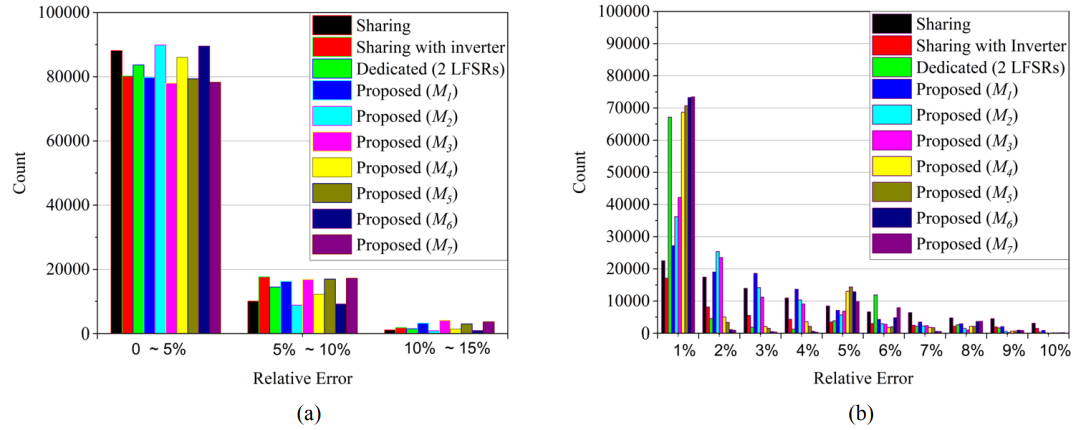Table 2. Comparison of the average stochastic computing correlation(AVG_SCC) and the error metrics; error distance average(AVG_ED), maximum error distance(MAX_ED), average absolute relative error (AVG_ABS_RE), and standard deviation (SD), and coefﬁcient variability (CV) between the various conventional stochastic methods (i.e., dedicated, sharing, and inverter) and the proposed method

 Various SNG Dedicated Sharing Inverter Proposed design $M_1$ $M_2$ $M_3$ $M_4$ $M_5$ $M_6$ $M_7$ $M_{AVG}$ AVG_SCC 0.042 1 -0.921 0.912 0.688 0.609 0.274 0.239 0.164 0.141 0.432 SC adder AVG_ED 5.678 4.768 6.236 6.281 4.463 6.584 5.090 6.351 4.471 6.506 5.678 MAX_ED 29 26 33 33 21 37 25 31 21 35 29 AVG_ABS_RE 0.027 0.024 0.029 0.031 0.022 0.033 0.025 0.032 0.022 0.032 0.028 SD 0.027 0.027 0.028 0.034 0.024 0.035 0.027 0.033 0.025 0.034 0.030 SC multiplier AVG_ABS_RE 0.189 0.341 0.646 0.267 0.206 0.191 0.158 0.155 0.104 0.158 0.184 SD 0.275 0.271 0.403 0.224 0.190 0.185 0.227 0.233 0.219 0.266 0.226

Table 3. Comparison of hardware metrics between the accurate and SC multipliers using various stochastic number generation methods in 45-nm technology

 Accurate SC with various SNG Binary Wallace tree Behavior Conventional method Proposed method Dedicated Sharing Inverter $M_1$ $M_2$ $M_3$ $M_4$ $M_5$ $M_6$ $M_7$ $M_{AVG}$ ASIC Area (µm$^{2}$) 376.6 454.2 409.4 350.9 289.7 286.9 289.3 289.7 289.7 289.5 289.5 290.0 290.0 289.7 Power (µW) 180.0 265.6 209.5 35.1 23.2 23.8 23.6 23.2 23.2 27.4 27.5 23.5 23.5 24.6

For a comparison with an application specific integrated circuit (ASIC), the area and power of the stochastic multiplier and accurate multipliers (i.e., binary, Wallace tree, and behavior multipliers) were analyzed using the 45-nm Nangate library in Design Compiler (23,24). The results are summarized in Table 3. As shown, the proposed design requires a smaller area and lower power than the accurate multiplier. For example, only 1/11 of power is required by the proposed design compared with the Wallace tree multiplier. In addition, the proposed design enables an approximately 25% reduction in the total area compared to the behavior multiplier

## IV. EDGE DETECTION WITH A PROPOSED STOCHASTIC COMPUTING

### 1. Basic Structures

We applied this technique to edge detection to clarify the effect. Edge detection algorithms such as Sobel can generate acceptable results in addition to offering relative simplicity and error tolerance; hence, they have been widely used in image processing. In general, the basic edge detection algorithm uses the horizontal and vertical gradients of an image to calculate based on the first- or second-order derivative of the digital level image. The gradient of an image, ∇f(x, y), in point (x, y) is defined as follows:

$\nabla f(x, y)=[\partial f / \partial x, \partial f / \partial y]=[G x, G y]$

The magnitude of this vector, which is described by ∇$f$, can reduce the noise effect owing to the smoothing operation by averaging the image gradient values, and the following methods are typically used for absolute value approximation:

$\operatorname{mag}(\nabla f)=\sqrt{G_{x}^{2}+G_{y}^{2}}=|G x|+|G y|$

The Sobel edge detection algorithm obtains an image gradient by calculating the partial derivatives x and y per pixel position that can be obtained from different masks, which are the horizontal mask ($Gx$) and vertical mask ($Gy$), around the pixel (25). In the Sobel algorithm, given a picture, for each pixel (i, j), the value ∇f is calculated from the brightness of the pixels, including (i-1, j-1), (i-1, j), (i-1, j+1), (i-1, j), (i+1, j), (i+1, j-1), (i+1, j), and (i+1, j+1):

$G_{x}=\left(\mid\left(x_{i+1, j-1}+2 x_{i+1, j}+x_{i+1, j+1}\right)+\left(x_{i-1, j-1}+2 x_{i-1 j}+x_{i-1, j+1}\right)\right) \mid$ $G_{y}=\left(\left|\left(y_{i-1, j-1}+2 y_{i-1, j}+y_{i+1, j-1}\right)+\left(y_{i-1, j+1}+2 y_{i, j+1}+y_{i+1 j+1}\right)\right|\right)$

where $x_{i,j}$ and $y_{i,j}$ denote the brightness of pixel (i, j). When mag(∇$f$) is large, pixel (i, j) can detect an edge.

### 1. Basic Structures

The image quality and design specifications were analyzed and compared between an accurate Sobel edge detection method (i.e., accurate adder, subtractor, multipliers, and absolute unit) and the stochastic edge detection method using various SNGs. Because the Sobel edge detection requires 16 SNs, we used 16 LFSRs in the dedicated method and only one LFSR in the other methods (i.e., sharing, inverter, and the proposed method) (15). In Fig. 5, the accurate Sobel edge detection circuit is shown in (a) and the stochastic computing edge detection circuit in (b) (15).

Fig. 5. Sobel edge detection circuit (a) accurate operators, (b) stochastic operators (15).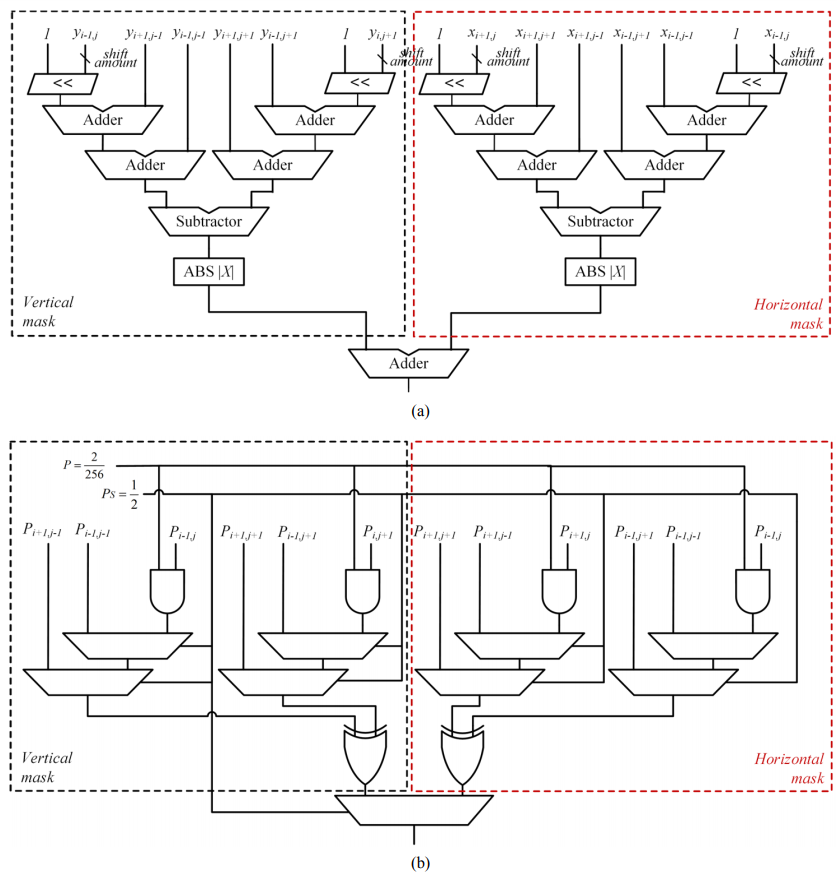The Sobel edge detection was designed with Verilog HDL and analyzed using an FPGA device, DE1-SoC board, and Cyclone V (5CSEMA4F31C6) from Terasic (26). In addition, the designs were synthesized using a 45-nm Nangate open-cell library in Design Compiler for hardware metrics comparison in ASIC. Fig. 6 shows the original image of 512×512 pixels and the results obtained using various edge detection methods. The method using accurate operators is shown in (b), and the stochastic operation results are shown in (c-k), which are the conventional methods (c, d, and e) and the proposed method (f-i). In conventional methods, sharing method and inverter methods use a single RNG for Sobel edge detection input. However, the dedicated method uses 16 RNGs to generate SNs for the 16 inputs of the Sobel edge detection, which can give accurate results by using more SNGs. As shown, the images obtained using the proposed SC technique is better than those by the other stochastic methods and is similar to the image obtained by the accurate edge detection. Table 4 shows the hardware design metrics and error metrics after the synthesis of the Sobel edge detection technique using an accurate design and the various stochastic designs including the proposed method. As shown, up to 65% of logic utilizations and 63% of power were saved on average in the FPGA compared with the accurate edge detection. When compared with the dedicated LFSR method, the proposed design average can achieve up to a 46% area reduction and 71% power reduction in the ASIC. The relative power signal noise ratio (PSNR) and the root-mean-square error (RMSE) of the proposed method average were 14.83 and 42.05 for the five sample images, respectively. The percentage difference between the images of the proposed design's average with respect to the image after an accurate Sobel edge detection was approximately 12%, which shows the high accuracy of the proposed design. Furthermore, the proposed design average can enhance the PSNR by 18%, reduce the RMSE by 21%, and decrease the percentage difference between images by 25% compared with the sharing and sharing with inverter methods.

Fig. 6. Original image and images obtained using various edge detection techniques (a) original image, (b) accurate Sobel edge detection, (c) stochastic Sobel edge detection by sharing the LFSR, (d) stochastic Sobel edge detection using an inverter, (e) stochastic Sobel edge detection using multiple LFSRs, and the proposed stochastic edge detection using (f) $M_{1}$, (g) $M_{2}$, (h) $M_{3}$, (i) $M_{4}$, (j) $M_{5}$, (k) $M_{6}$, and (l) $M_{7}$ in Fig. 2.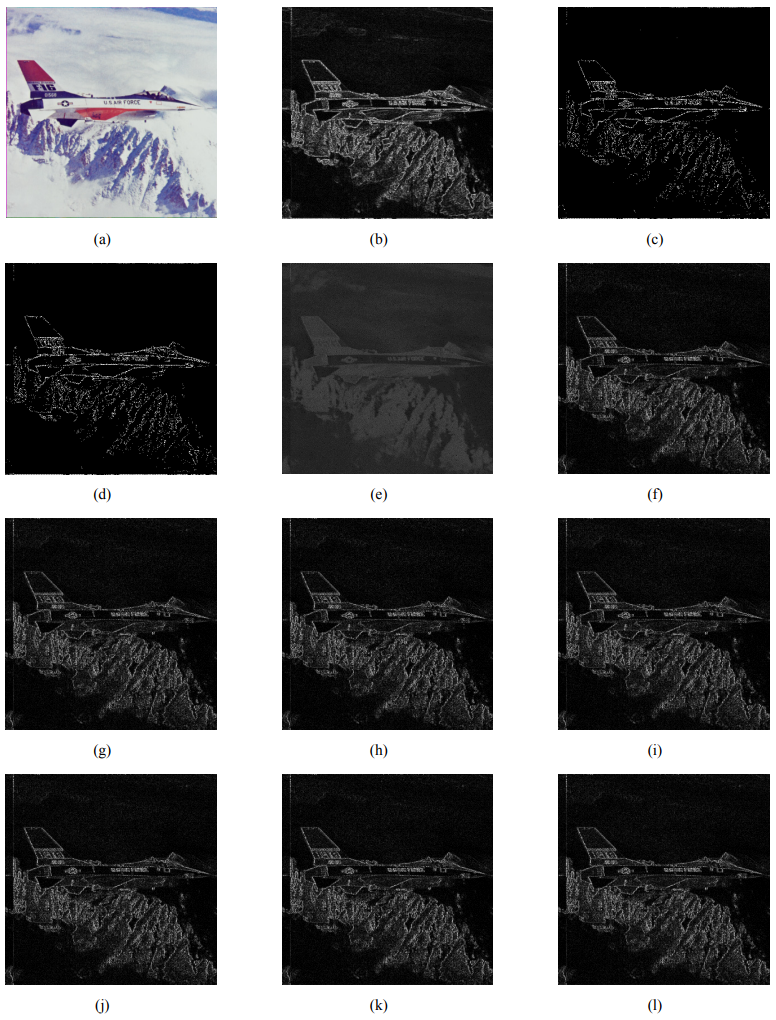Table 4. Comparison in hardware metrics and image quality between the accurate and stochastic Sobel edge detection with various benchmark images

 ACC. Conventional method Proposed method Sharing  Inverter  Dedicated  $M_1$ $M_2$ $M_3$ $M_4$ $M_5$ $M_6$ $M_7$ $M_{AVG}$ FPGA Total register 24 32 32 52 32 32 32 32 32 32 32 32 Logic utilization (<32070) 60 21 21 56 21 21 21 21 21 21 21 21 Power (mW) 2.77 0.83 1.08 1.21 1.08 1.14 1.14 1.17 1.17 0.84 0.84 1.05 ASIC Area (µm$^{2}$) 846.1 317.6 316.8 598.7 317.6 320.7 320.7 322.1 322.1 320.0 320.0 320.5 Power (µW) 649.8 26.3 25.4 66.7 26.3 26.2 26.3 26.0 26.0 26.2 26.2 26.2 PSNR (dB) Airplane - 13.5 13.4 15.8 16.0 16.0 16.0 16.0 16.0 16.1 16.1 16.0 Lenna - 12.2 12.2 15.2 13.9 13.9 13.9 13.9 13.9 14.0 14.0 13.9 Pepper - 12.6 12.6 15.8 15.4 15.4 15.4 15.4 15.4 15.5 15.5 15.4 Sailboat - 10.0 10.0 13.2 12.2 12.2 12.2 12.3 12.3 13.4 13.4 12.6 Tiffany - 14.3 16.2 15.3 16.2 16.2 16.2 16.2 16.2 16.2 16.2 16.2 Average - 12.5 12.5 15.1 14.8 14.8 14.8 14.8 14.8 15.0 15.0 14.8 RMSE Airplane -- 47.2 47.2 35.8 38.9 38.9 38.9 38.9 38.9 36.7 36.7 38.3 Lenna - 54.0 54.2 38.3 44.5 44.5 44.5 44.5 44.5 42.0 42.0 43.8 Pepper - 52.4 52.2 36.0 42.2 42.2 42.2 42.1 42.1 39.7 39.7 41.4 Sailboat - 69.8 69.8 48.3 54.0 54.0 54.0 53.9 53.9 51.1 51.1 53.1 Tiffany - 42.8 42.7 37.9 34.2 34.2 34.2 34.2 34.2 32.2 32.2 33.6 Average - 53.2 53.2 39.3 42.8 42.8 42.8 42.7 42.7 40.3 40.3 42.1 Percentage difference between images (%) Airplane - 13.0 13.0 12.6 10.2 10.2 10.2 10.2 10.2 9.2 9.2 9.9 Lenna - 17.3 17.4 13.8 13.9 13.9 13.9 13.9 13.9 12.6 12.6 13.5 Pepper - 16.5 16.5 11.3 12.6 12.6 12.6 12.6 12.6 11.3 11.3 12.2 Sailboat - 21.9 21.9 15.3 16.2 16.2 16.2 16.1 16.1 14.8 14.8 15.7 Tiffany - 13.3 13.3 14.5 10.1 10.1 10.1 10.1 10.1 9.1 9.1 9.8 Average - 16.4 16.4 13.5 12.6 12.6 12.6 12.6 12.6 11.4 11.4 12.3

## IV. CONCLUSIONS

Herein, we propose an unprecedented SNG for a compact and accurate stochastic operation. The proposed method involved the sharing of random number sources by output changing based on a fully connected cube network. Furthermore, the method can reduce the size of SNGs significantly. The experimental results indicated that our proposed designs were smaller than the previous design without sacrificing the accuracy of stochastic multiplication significantly. For example, the proposed stochastic multiplier could an area reduction of up to 37%, and power reduction by 91% compared with the Wallace tree multiplier. Compared with the absolute relative error of the conventional stochastic multiplier based on the sharing method, that of the proposed design's average was reduced by up to 72%. To verify the applicability of the proposed design for the real world, we implemented an image processing application. The stochastic Sobel edge detection based on the proposed design offered significant advantages in terms of area and power over the exact method and enhanced the image quality compared with conventional stochastic designs. Therefore, the proposed design could be applied to energy-efficiency hardware for system on chip (SoC)-like image processing applications by exploiting the imprecision tolerance. In the future, we plan to apply the proposed design to LDPC.

### ACKNOWLEDGMENTS

This work was supported by the NRF of Korea funded by the MSIT under Grant NRF-2019M3F3A1A02072093 (Intelligent Semiconductor Technology Development Program). The EDA Tool was supported by the IC Design Education Center.

### REFERENCES

1
Tong J. Y. F., Nagle D., Rutenbar R. A., June 2000, Reducing power by optimizing the necessary precision/range of ﬂoating-point arithmetic, IEEE Transactions on Very Large Scale Integration (VLSI) Systems, Vol. 8, No. 3, pp. 273-2862
Sampson A., Dietl W., Fortuna E., Gnana-pragasam D., Ceze L., Grossman D., June 2011, Enerj: Approximate data types for safe and general low-power computation, ACM SIGPLAN Notices, Vol. 46, No. 6, pp. 164-1743
Han J., Orshansky M., May 2013, Approximate computing: An emerging paradigm for energy-efficient design, in Proc. of the 18th IEEE European Test Symposium (ETS), pp. 1-64
Agrawal A., Choi J., Gopalakrishnan K., Gupta S., Nair R., Oh J., Prener D. A., Shukla S., Srinivasan V., Sura Z., Oct 2016, Approximate computing: Challenges and opportunities, in Proc. of 2016 IEEE International Conference on Rebooting Computing (ICRC), pp. 1-85
Gaines B. R., 1969, Stochastic computing systems, Advances in Information Systems Science, Vol. 2, pp. 37-1726
Qian W., Li X., Riedel M. D., Bazargan K., Lilja D. J., Jan 2011, An architecture for fault-tolerant computation with stochastic logic, IEEE Transactions on Computers, Vol. 65, No. 1, pp. 93-1057
Alaghi A., Hayes J. P., 2013, Survey of stochastic computing, ACM Transactions on Embedded Computing Systems (TECS), vol. 12(2s), Vol. no. 92, pp. 1-198
Alaghi A., Hayes J. P., Mar 2014, Fast and accurate computation using stochastic circuits, in Proc. of Design, Automation & Test in Europe Conference & Exhibition (DATE), pp. 1-49
Alaghi A., Hayes J. P., Apr 2015, Dimension reduction in statistical simulation of digital circuits, in Proc. of the Symposium on Theory of Modeling & Simulation (TMS DEVS), pp. 1-810
Lee V. T., Alaghi A., Ceze L., 2018, Correlation manipulating circuits for stochastic computing, in Proc. of Design, Automation & Test in Europe Conference & Exhibition (DATE), pp. 1417-142211
Alaghi A., Qian W., Hayes J. P., Aug 2018, The promise and challenge of stochastic computing, IEEE Transactions on Computer-Aided Design of Integrated Circuits and Systems, Vol. 37, No. 8, pp. 1515-153112
Alaghi A., Hayes J. P., Nov 2013, Exploiting correlation in stochastic circuit design, in Proc. of the 31st International Conference on Computer Design (ICCD), pp. 39-4613
Parhi K. K., 2017, Analysis of stochastic logic circuits in unipolar, bipolar and hybrid formats, in Proc. of International Symposium on Circuits and Systems (ISCAS), pp. 1-414
Ichihara H., Ishii S., Sunamori D., Iwagaki T., Inoue T., Oct 2014, Compact and accurate stochastic circuits with shared random number sources, in Proc. of International Conference on Computer Design (ICCD), pp. 361-36615
Joe H., Kim Y., 2019, Novel stochastic computing for energy-efﬁcient image processors, Electronics, Vol. 8, No. 6, pp. 449-46216
Yang M., Li B., Lilja D. J., Yuan B., Qian W., 2018, Towards theoretical cost limit of stochastic number generators for stochastic computing., in Proc. of the IEEE Computer Society Annual Symposium on VLSI (ISVLSI’18), pp. 154-15917
Li P., Lilja D. J., Qian W., Bazargan K., Riedel M. D., Mar 2014, Computation on Stochastic Bit Streams Digital Image Processing Case Studies, IEEE Transactions on Very Large Scale Integration (VLSI) Systems, Vol. 22, No. 3, pp. 449-46218
Alaghi A., Cheng Li., Hayes J. P., May 2013, Stochastic circuits for real-time image-processing applications, in Proc. of the 50th Annual Design Automation Conference (DAC), pp. 1-619
Akyildiz I. F., Wang X., Mar 2005, Wireless mesh networks: a survey, IEEE Communications magazine, pp. 445-48720
Esfahanian A. H., 1989, Generalized measures of fault tolerance with application to n-cube networks, IEEE Transactions on Computers, pp. 1586-159121
Duato J., Yalamanchili S., Ni L., 2013, Inter-connection networks, Morgan Kaufmann23
Nangate: 45nm Open Cell Library, https://www. silvaco.com/products/nangate/FreePDK45_Open_Cell_Library24
Design Compiler ver.M-2016.12-SP5-5, https:// www.synopsys.com/25
Sobel I., Feldman G., 1968, A 3x3 isotropic gradient operator for image processing, a talk at the Stanford Artificial Project, pp. 271-27226
DE1-SoC User manual 1.2.2, http://www.terasic.com/## Author

Hounghun Joe received B.S. and M.S. degree in computer engineering from Kwangwoon University, Seoul, Korea, in 2018 and 2020, respectively.

His research interests include embedded system designs, SoC designs, and low-power SoC designs.

Youngmin Kim received a BSc in electrical engineering from Yonsei University, Seoul, Korea, in 1999, and an MSc and a PhD in electrical engineering from the University of Michigan, Ann Arbor, in 2003 and 2007, respectively.

He held a senior engineering position at Qualcomm in San Diego, CA.

He is currently an Associate Professor at Hongik University, Seoul, South Korea.

Prior to joining Hongik University, he was with the School of Computer and Information Engineering at Kwangwoon University, Seoul, South Korea, and the School of Electrical and Computer Engineering at the Ulsan National Institute of Science and Technology (UNIST), Ulsan, South Korea.

His research interests include embedded systems, variability-aware design methodologies, design for manufactur-ability, design and technology co-optimization methodologies, and low-power and 3D IC designs.